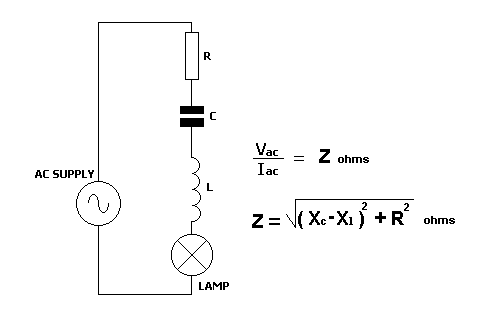Home > Electronic Tutorials > Resistance and Impedance in AC Circuit > R, C and L in an AC Circuit Tutorial

Resistance and Impedance in AC Circuit

# R, C and L in an AC Circuit TutorialThe resistor, the capacitor and the coil all oppose the flow of ac current. Their combined opposition is called impedance, Z.

Ohm's Law can be applied, as shown in the top formula. The higher the impedance, the lower the current.

The resistor has resistance. The capacitor has capacitive reactance. The coil has inductive reactance. All of these values are measured in ohms.

However, impedance is not calculated by adding these values.

The bottom formula must be used.

If you are familiar with Pythagoras, this is another application of it.

When coupling one device to another, such as an amplifier to a loudspeaker, the output impedance of the amplifier and  the input impedance of the speaker must be the same, to give optimum transfer of power from one to the other.